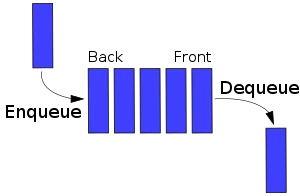# Programming - C Queues using ArraysToday’s subject are Queues in C Language using Array Implementation. We will talk about the basic functions that add (enqueue), remove (dequeue) an Item and we will also print the queue. So, without further do, let’s get started.

# Queue Array Implementation:

An Queue is an Data Structure that implements the FIFO (first in first out) way of getting information. So, we insert an Item in the front and get an item from the end. We define a Queue like all other Data Structures as an struct in C that contains (because we use Array implementation):

• an static Array that contains the Queue Items
• the front index of the Queue in the Array
• the rear index of the Queue in the Array

We could also include the capacity of the Array if we make it pseudodynamic.

To make it simple I will only talk about Integer Arrays. Having a struct Array you can then create more advanced behavior including more informations like priority numbers etc. that can make your Queue a Priority Queue in no time, having more advanced Code of course.

The struct will look like this:

``typedef struct Queue{  ``
``    int q[N];  ``
``    int front;``
``    int rear;  ``
``}Queue;``

The front and rear will be initialized as -1, that means that the queue is empty.

The functions that use a Queue will have an struct variable of type Queue and we will use this variable for doing stuff like adding, removing an Item or printing the Queue. Because we are using an Array the functions are pretty simple. We will pass the address of the struct variable and do everything inside of functions. To keep things simple I will add an item to the rear index and take it from the front index.

For adding an Item we have to check if queue is full that means we can’t add a Item. We, also have to check if queue is empty to set front and rear to 0 and afterwards add the item to the rear index. And the basic case has to increment our rear index by 1 and add an item to rear index. The last two can be done using one line of adding code.

So, our Code looks like this:

``void enqueue(Queue *Q, int val){``
`` // check if full``
`` if( (Q->rear - Q->front + 1) == N){``
``     printf("Queue is Full!\n");``
``     return;``
`` }``
`` // check if empty``
`` else if(Q->front == -1){``
``     Q->front = Q->rear = 0;``
`` }``
`` // basic case``
`` else{``
``     // simply increment rear index``
``     Q->rear++;       ``
`` }``
`` // add item to rear of Queue``
`` Q->q[Q->rear] = val;``
``}``

# Removing (Dequeue):

When removing an item we get it from the front index and return it. We can’t search like we used to do and we also have to check if queue is empty that means we can’t delete anything by checking if front is -1 again and returning -1. A special case is having only one item inside were we than have to set front and rear to -1. The normal case is simply returning front after incrementing front by 1. So, our queue can now have the front index be different and not only 0!

The Code looks like this:

``int dequeue(Queue *Q){``
`` int val;``
`` // check if empty``
`` if(Q->front == -1){``
``     return -1;``
`` }``
`` // check if one item inside``
`` else if(Q->front == Q->rear){``
``     val = Q->q[Q->front];``
``     Q->front = Q->rear = -1;``
`` }``
`` // basic case``
`` else{``
``     val = Q->q[Q->front];``
``     Q->front++;``
`` }``
`` //return the value ``
`` return val; ``
``}``

# Printing:

Printing is simple we will simply not put the Queue as a pointer, but by its own as a parameter and use the dequeue function as long as it doesn’t return -1. That way the information doesn’t get lost.

We could also create a for loop from rear to front like this:

``void print(Queue Q){ ``
``    int i;  ``
``    // check if empty  ``
``    if(Q.front == -1){  ``
``        printf("Queue is Empty!\n");  ``
``        return;  ``
``    } ``
``     for(i = Q.rear; i>=Q.front ; i--){  ``
``        printf("%d ", Q.q[i]);  ``
``     }  ``
``     printf("\n"); ``
``} ``

But, I think it makes more sense this way:

``void print(Queue Q){``
`` int val;``
`` // check if empty``
`` if(Q.front == -1){``
``     printf("Queue is Empty!\n");``
``     return;``
`` }``
`` // call dequeue as long as there are items``
`` while((val= dequeue(&Q)) != -1){``
``     printf("%d ", val);``
`` }   ``
`` printf("\n");``
``}``

# Code:

Last for today here a Code that uses those functions so that you know how to set them up. And to point it out really quick the N from the queue’s array is defined with hashtag define N 15 or something after the library declaration, but I think I already showed it some posts before.

``int main(){``
`` // initialize Queue``
`` Queue Q;``
`` Q.front = Q.rear = -1;``
`` print(Q);``
`` // add 5 to queue``
`` enqueue(&Q, 5);``
`` print(Q);``
`` // add 10 to queue``
`` enqueue(&Q, 10);``
`` print(Q);``
`` // remove from queue``
`` int val;``
`` val = dequeue(&Q);``
`` if(val == -1){``
``     printf("Queue is Empty!\n");``
`` }``
`` else{``
``     printf("%d\n", val);``
`` }``
`` print(Q);   ``
``}``

Next up: C Stacks using Arrays. Hope you enjoyed it! :)
I also would like to point out that woz.software will upload some of my Code in different Language implementation.
Be sure to take a look at his stuff too!

And a little showcase more. After uploading the Java Exercise Solution I will do all this stuff that I did with C Data Structures in Java too!

H2
H3
H4
3 columns
2 columns
1 column